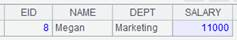• 集算器
教程
函数参考
用户参考
外部库使用指南
数据文件工具用户参考
DQL教程
集群管理器使用说明
SPL命令执行器用户参考
• 润乾报表
教程
填报教程
分析教程
报表中心教程
用户参考
程序员参考
高级指南
通用查询
DQL部署集成手册
• 易明建模
用户参考
json参数说明
• 润乾官网

# groups()

## A.groups()

A.groups(x:F,…;y:G,…)

 @o 只和相邻对比，相当于归并，结果集不再排序 @n x取值为分组序号，可直接定位，与@o互斥 @u 结果集不再按x排序；与@o/@n互斥 @i x是布尔表达式，如果x的结果为true则开始新的一组。此时只有一个x @m 数据量大的复杂运算中，并行计算提升性能，计算次序不确定，与@o/@i互斥 @0 丢弃x的计算结果为空的组 @h 用于分段有序的数据，可提高分组效率 @t 当A的返回值为空，则返回一个保留数据结构的空序表 @z(…;…;n) 并行计算时按分组拆分，多线程共享结果集，此时不会动态调整HASH空间，n为HASH空间长度，可用缺省值 @e 返回y的结果组成的序表。其中x为A的字段名，y是A的函数，y的计算结果要求是A的一条记录，当y是聚合函数时仅支持maxp/minp/top@1

 A 序列 x 分组表达式 F 结果序表的字段名 G 结果序表中的汇总字段名 y y是A的函数。当y为聚合函数时:仅支持sum/count/max/min/top/avg/iterate /icount/median/maxp/minp，使用iterate(x,a;Gi,…)时不能有参数Gi。当y为非聚合函数时:只对每组第1条计算。

 A 1 =demo.query("select * from SCORES where CLASS = 'Class one'")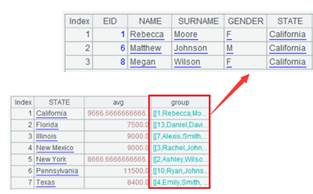2 =A1.groups(STUDENTID:StudentID;sum(SCORE):TotalScore)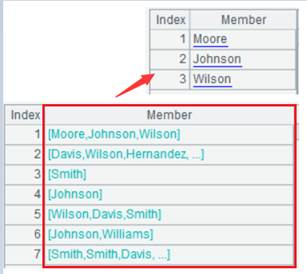单字段分组 3 =demo.query("select * from SCORES") 4 =A3.groups(CLASS:Class,STUDENTID:StudentID;sum(SCORE):TotalScore)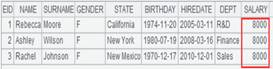多字段分组 5 =A3.groups@m(STUDENTID:StudentID;sum(SCORE):TotalScore) 数据量大时提升性能 6 =A3.groups@o(STUDENTID:StudentID;sum(SCORE):TotalScore)只和相邻的对比归并，结果集不排序 7 =demo.query("select * from STOCKRECORDS where STOCKID<'002242'") 8 =A7.groups@n(if(STOCKID=="000062",1,2):StockID;sum(CLOSING):TotalPrice)x取值为分组序号 9 =demo.query("select * from EMPLOYEE") 10 =A9.groups@u(STATE:State;count(STATE):TotalScore) 结果集不按分组字段排序 11 =A9.groups@i(STATE=="California":IsCalifornia;count(STATE):count)遇到STATE=="California"则开始新的分组 12 =A3.groups(CLASS:Class,STUDENTID:StudentID;iterate(~~*2,10): Score1)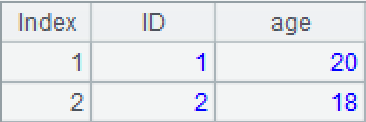分组的每组内进行iterate运算 13 =file("D:\\Salesman.txt").import@t()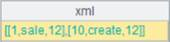14 =A13.groups@0(Gender:Gender;sum(Age):Total)丢弃Gender为空的组 15 =file("D:/emp10.txt").import@t() 数据文件emp10.txt中，每10条数据根据DEPT进行了一次排序16 =A15.groups@h(DEPT:dept;sum(SALARY):bouns) A15是以DEPT分段有序的数据，使用@h选项提高分组效率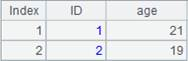17 =A1.groups(STUDENTID:StudentID;SUBJECT,sum(SCORE):SUMSCORE) 非聚合函数计算第一条数据,English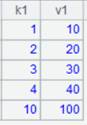18 =demo.query("select * from SCORES where CLASS = 'Class three'") 返回空序表 19 =A18.groups@t(STUDENTID:StudentID;sum(SCORE):TotalScore) 返回保留数据结构的空序表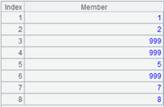A 1 =demo.query("select * from SCORES") 2 =A1.groups@z(STUDENTID:StudentID;sum(SCORE):TotalScore;5) 并行计算时按组拆分，HASH空间长度为5

 A 1 =demo.query("select EID,NAME,GENDER,DEPT,SALARY from employee") 2 =A1.groups(DEPT;minp(SALARY))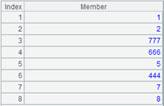执行聚合函数minp()后返回A3的记录 3 =A1.groups@e(DEPT;minp(SALARY))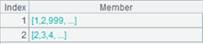返回由minp(SALARY)结果记录构成的序表

A.group(xi,…)

## ch.groups()

ch.groups(x:F,…;y:G…;n)

 @n x取值为分组序号，可直接定位 @u 结果集不再按x排序；与@n互斥

 ch 管道 x 分组表达式，x:F省略表示针对全集聚合，此时“；”不可省略。 F 结果序表的字段名 y ch的聚合函数，仅支持sum/count/max/min/top /avg/iterate，使用iterate(x,a;Gi,…)时不能有参数Gi。 G 结果序表中的汇总字段名

 A 1 =demo.cursor("select * from EMPLOYEE ") 2 =channel() 创建管道 3 =channel() 创建管道 4 =channel() 创建管道 5 =channel() 创建管道 6 =A1.push(A2,A3,A4,A5) 将游标A1中的数据推送到管道A2、A3、A4和A5，此时数据不会立即被推送到管道。 7 =A2.groups(;sum(SALARY):TotalSalary) 省略x:F求所有员工的总工资 8 =A3.groups(DEPT:dept;sum(SALARY):TotalSalary) 指定字段DEPT分组，并按照指定字段排序 9 =A4.groups@n(if(GENDER=="F",1,2):SubGroups;sum(SALARY):TotalSalary) x取值为分组序号，GENDER等于“F”的分到第一组，否则分到第二组，并且每个组聚合 10 =A5.groups@u(STATE:State;count(STATE):count) 结果集不按分组字段排序 11 = A1.select(month(BIRTHDAY)==2) 12 =A11.fetch() 游标A11添加了计算。 13 =A2.result()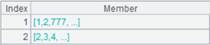14 =A3.result()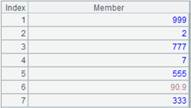15 =A4.result()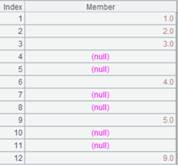16 =A5.result()## cs.groups()

cs.groups(x:F,…;y:G…;n)

 @c 分机独立执行，结果集拼成集群内表，保持游标分布，可用作集群维表

 cs 集群游标记录 x 分组表达式，x:F省略表示针对全集聚合，此时“；”不可省略。 F 结果序表的字段名 y cs的聚合函数，仅支持sum/count/max/min/top/avg/iterate，使用iterate(x,a;Gi,…)时不能有参数Gi。 G 结果序表中的汇总字段名 n 最大分组数，超过n时停止执行，用于分组数非常大时，防止内存溢出。并且最后的分组数不是确切数n，可能会比n大一些。

 A 1 =file("emp1.ctx","192.168.0.111:8281") emp1.ctx数据文件内容如下：2 =A1.open() 打开集群组表 3 =A2.cursor() 返回集群游标 4 =A3.groups(Dept:dept;count(Name):count) 根据DEPT分组，然后聚合A 1 [192.168.0.110:8281,192.168.18.143:8281] 2 =file("emp.ctx":[1,2], A1) 3 =A2.open() 打开集群组表 4 =A3.cursor() 获取集群游标 5 =A4.groups(GENDER:gender;sum(SALARY):totalSalary) 根据GENDER分组后再聚合，返回序表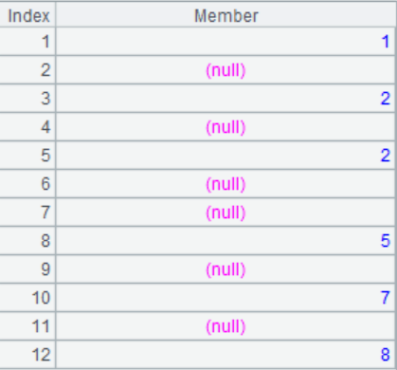6 =A3.cursor() 7 =A6.groups@c(GENDER:gender;sum(SALARY):totalSalary).dup() 游标保持分布，返回集群内表## cs.groups()

cs.groups(x:F,…;y:G…)

 @n x取值为分组序号，可直接定位，n为事先设定的总组数，可以直接先生成空间 @u 结果集不再按x排序；与@n互斥 @o 只和相邻对比，相当于归并，结果集不再排序 @i x是布尔表达式，如果x的结果为true则开始新的一组。此时只有一个x。 @h 用于分段有序的数据，可提高分组效率 @0 丢弃x的计算结果为空的组 @t 当游标中的数据返回值为空，则返回一个保留数据结构的空序表 @z(…;…;n) 并行计算时按分组拆分，多线程共享结果集，此时不会动态调整HASH空间，n为HASH空间长度，可用缺省值 @e 返回y的结果组成的序表。其中x为cs的字段名，y是cs的函数，y的计算结果要求是cs中的一条记录，当y是聚合函数时仅支持maxp/minp/top@1

 cs 游标记录 x 分组表达式，x:F省略表示针对全集聚合，此时“；”不可省略。 F 结果序表的字段名 y cs的聚合函数，仅支持sum/count/max/min/top/avg/iterate/minp/maxp，使用iterate(x,a;Gi,…)时不能有参数Gi。 G 结果序表中的汇总字段名

 A 1 =demo.cursor("select * from SCORES where CLASS = 'Class one'") 2 =A1.groups(;sum(SCORE):TotalScore)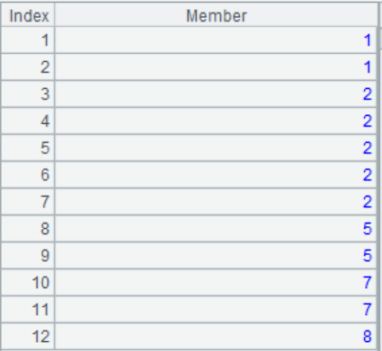省略x:F求所有学生的总成绩 3 =demo.cursor("select * from FAMILY") 4 =A3.groups(GENDER:gender;sum(AGE):TotalAge)指定字段分组，并按照指定字段排序 5 =demo.cursor("select * from STOCKRECORDS where STOCKID<'002242'") 6 =A5.groups@n(if(STOCKID=="000062",1,2):SubGroups;sum(CLOSING):ClosingPrice)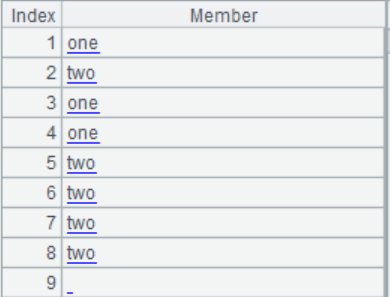x取值为分组序号，STOCKID等于“000062”的分到第一组，否则分到第二组，并且每个组聚合 7 =demo.cursor("select * from EMPLOYEE") 8 =A7.groups@u(STATE:State;count(STATE):Total) 结果集不按分组字段排序 9 =demo.cursor("select * from EMPLOYEE") 10 =A9.groups@o(STATE:State;count(STATE):Total) 只和相邻的对比，结果不排序11 =demo.cursor("select * from EMPLOYEE") 12 =A11.groups@i(STATE=="California":IsCalifornia;count(STATE):count)遇到STATE=="California"则开始新的分组 13 =file("D:/emp10.txt").cursor@t() 数据文件emp10.txt中，每10条数据根据DEPT进行了一次排序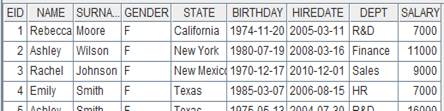14 =A13.groups@h(DEPT:DEPT;sum(SALARY):bouns) A13是以DEPT分段有序的数据，使用@h选项提高分组效率15 =demo.query("select  * from employee") 16 =A15.cursor@m(3) 返回多路游标 17 =A16.groups(STATE:state;sum(SALARY):salary) 多路游标使用groups并行分组A 1 =demo.cursor("select * from SCORES where CLASS = 'Class three'") 2 =A1.groups@t(STUDENTID:StudentID;sum(SCORE):TotalScore) 返回保留数据结构的空序表3 =demo.cursor("select * from DEPT") DEPT表数据内容如下：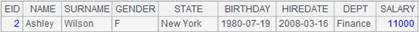4 =A3.groups@0(FATHER) 丢弃计算结果为空的组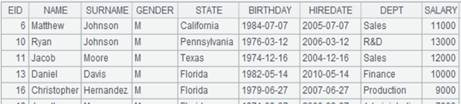A 1 =demo.cursor("select * from SCORES") 2 =A1.groups@z(STUDENTID:StudentID;sum(SCORE):TotalScore;5) 并行计算时按组拆分，HASH空间长度为5

 A 1 =demo.cursor("select * from SCORES") 2 =A1.groups@e(SUBJECT;maxp(SCORE))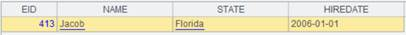返回由maxp(SCORE)结果记录构成的序表

## T.groups()

T. groups(x:F,…;y:G…;n)

 @n x取值为分组序号，可直接定位，n为事先设定的总组数，可以直接先生成空间 @u 结果集不再按x排序；与@n互斥 @o 只和相邻的对比，相当于归并，结果集不再排序 @i x是布尔表达式，如果x的结果为true则开始新的一组。此时只有一个x @h 用于分段有序的数据，可提高分组效率 @b 返回的结果集中只有聚合数据，没有分组列数据 @v 组表第一次加载时用列式，提升性能

 T 虚表 x 分组表达式，x:F省略表示针对全集聚合，此时；不可省略 F 结果序表的字段名 y 聚合函数，仅支持sum/count/max/min/top/avg/iterate，使用iterate(x,a;Gi,…)时不能有参数Gi G 结果序表中的汇总字段名 n 最大分组数，超过n时停止执行，用于分组数非常大时，防止内存溢出。并且最后的分组数不是确切数n，可能会比n大一些

 A 1 =create(file).record(["D:/file/pseudo/empT.ctx"]) 2 =pseudo(A1) 生成虚表 3 =A2.groups(DEPT:dept;avg(SALARY):AVG_SALARY) 将虚表记录分组，分组字段为DEPT，聚合方式为计算每组SALARY的平均值，结果返回序表，结果序表中的字段名称有dept、AVG_SALARY，结果根据dept排序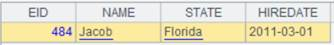4 =A2.groups@n(if(GENDER=="F",1,2):GenderGroup;avg(SALARY):AVG_SALARY) 将虚表记录根据GENDER是否为F分为两组，并计算每组的SALARY平均值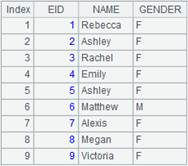5 =A2.groups@u(DEPT:dept;avg(SALARY):AVG_SALARY) 使用@u，分组结果不排序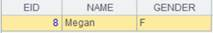6 =A2.groups@o(DEPT:dept;avg(SALARY):AVG_SALARY) 使用@o，只和相邻对比，结果集不排序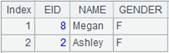7 =A2.groups@i(GENDER=="M":isMAN;count(EID):Count) 遇到GENDER=="M"则开始新的分组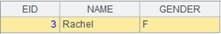8 =A2.groups@b(DEPT:dept;avg(SALARY):AVG_SALARY) 使用@b选项，结果集中只有聚合数据列，无分组数据列